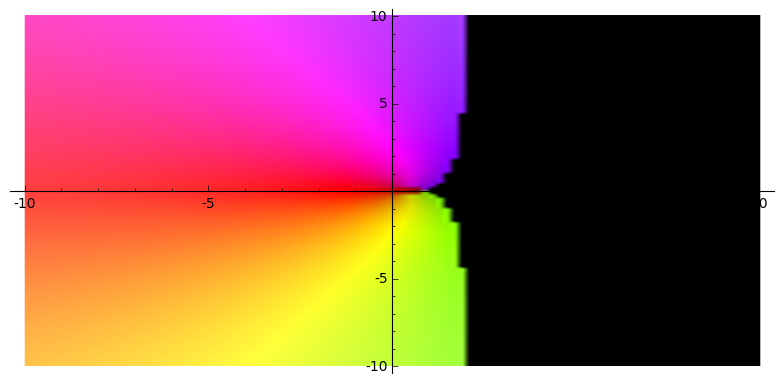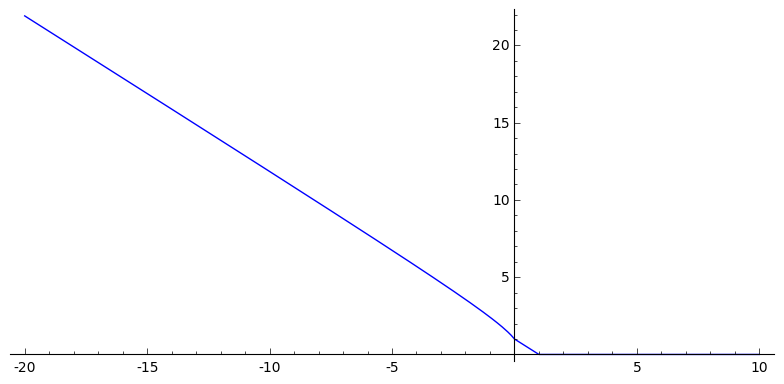# Revision history [back]

Not entirely sure why you assume v is complex and want to plot it when $v\in [-1,7]$.

Perhaps this is what you want to use (?).

complex_plot(lambda v: abs(matrix(CC, [[0,1,0,0],[-v,1-v,0,0],[0,-v,1-v,0],[0,0,1,0]]).eigenvalues()),(-10,10),(-10,10))


Sample output:Although, then you may want to drop the abs to get:Not entirely sure why you assume v is complex and want to plot it when $v\in [-1,7]$.

Perhaps this is what you want to use (?).use.

complex_plot(lambda v: abs(matrix(CC, [[0,1,0,0],[-v,1-v,0,0],[0,-v,1-v,0],[0,0,1,0]]).eigenvalues()),(-10,10),(-10,10))


Sample output:Although, then you may want to drop the abs to get:If you are just interested in the case where $v\in \mathbb{R}$ and want to plot the module of the eigenvalue then

plot(lambda v: abs(matrix(CC, [[0,1,0,0],[-v,1-v,0,0],[0,-v,1-v,0],[0,0,1,0]]).eigenvalues()),(x,-20,10))


will do the job.Update: Added case where $v\in[1,7]$.

Perhaps this is what you want to use.

complex_plot(lambda v: abs(matrix(CC, [[0,1,0,0],[-v,1-v,0,0],[0,-v,1-v,0],[0,0,1,0]]).eigenvalues()),(-10,10),(-10,10))


Sample output:Although, then you may want to drop the abs to get:If you are just interested in the case where $v\in \mathbb{R}$ and want to plot the module of the eigenvalue then

plot(lambda v: abs(matrix(CC, [[0,1,0,0],[-v,1-v,0,0],[0,-v,1-v,0],[0,0,1,0]]).eigenvalues()),(x,-20,10))


will do the job.Update: Added case where $v\in[1,7]$.$v\in\mathbb{R}$.